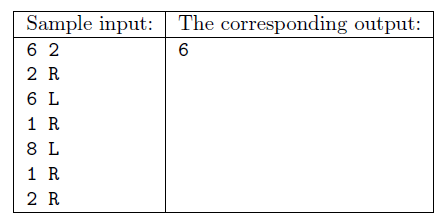Mathematical and Physical Journal
for High Schools
Issued by the MATFUND Foundation
 Already signed up? New to KöMaL?

#Problem S. 102. (November 2015)

S. 102. A certain robot is controlled by the following commands: it starts from position 0, then upon receiving 15 R and 20 L, for example, it makes 15 steps to the right then 20 steps to the left. The robot receives $\displaystyle N$ commands ($\displaystyle 1\le N\le 300\;000$). The step numbers appearing in these R/L commands are positive integers, and the maximum allowed distance of the robot from the origin is $\displaystyle 10^{9}$. You are also given a number $\displaystyle K$. Your task is to determine the number of positions on or over which the robot passed at least $\displaystyle K$ times.

Your program should read the values of $\displaystyle N$ and $\displaystyle K$ from the first line of the standard input, then the $\displaystyle a_i$, $\displaystyle c_i$ number-character pairs (separated by a space) from the following $\displaystyle N$ lines describing the motion of the robot. The first and only line of the standard output should contain the appropriate number of positions the robot visited.Scoring and bounds. You can get 1 point for a brief and proper documentation clearly describing your solution. Nine further points can be obtained provided that your program solves any valid input within 1 second of running time.

The source code of your program without the .exe or any other auxiliary files generated by the compiler but with a short documentation---also describing which developer environment to use for compiling the source---should be submitted in a compressed file s102.zip.

(10 pont)

Deadline expired on December 10, 2015.

### Statistics:

 26 students sent a solution. 10 points: Alexy Marcell, Bálint Martin, Csenger Géza, Fuisz Gábor, Gáspár Attila, Gergely Patrik, Hornák Bence, Janzer Orsolya Lili, Kiss Gergely, Máté Nagy, Mernyei Péter, Molnár-Sáska Zoltán, Németh 123 Balázs, Szakály Marcell, Tóth Márk Andor, Zalavári Márton, Zarándy Álmos. 9 points: Cseh Viktor. 8 points: 2 students. 5 points: 2 students. 1 point: 1 student. 0 point: 3 students.

Problems in Information Technology of KöMaL, November 2015